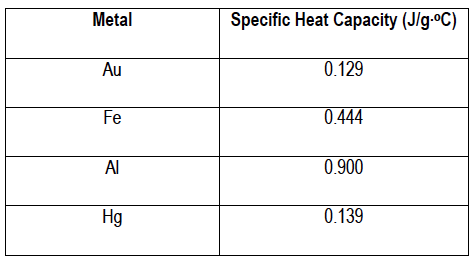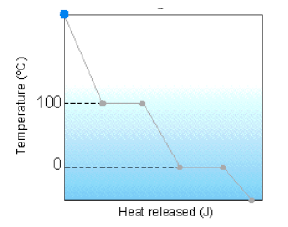Ch.6 - Thermochemistry WorksheetSee all chapters
 Ch.1 - Intro to General Chemistry 2hrs & 53mins 0% complete Worksheet Ch.2 - Atoms & Elements 2hrs & 40mins 0% complete Worksheet Ch.3 - Chemical Reactions 3hrs & 25mins 0% complete Worksheet BONUS: Lab Techniques and Procedures 1hr & 38mins 0% complete Worksheet BONUS: Mathematical Operations and Functions 47mins 0% complete Worksheet Ch.4 - Chemical Quantities & Aqueous Reactions 3hrs & 30mins 0% complete Worksheet Ch.5 - Gases 3hrs & 47mins 0% complete Worksheet Ch.6 - Thermochemistry 2hrs & 28mins 0% complete Worksheet Ch.7 - Quantum Mechanics 2hrs & 35mins 0% complete Worksheet Ch.8 - Periodic Properties of the Elements 1hr & 57mins 0% complete Worksheet Ch.9 - Bonding & Molecular Structure 2hrs & 5mins 0% complete Worksheet Ch.10 - Molecular Shapes & Valence Bond Theory 1hr & 31mins 0% complete Worksheet Ch.11 - Liquids, Solids & Intermolecular Forces 3hrs & 40mins 0% complete Worksheet Ch.12 - Solutions 2hrs & 17mins 0% complete Worksheet Ch.13 - Chemical Kinetics 2hrs & 22mins 0% complete Worksheet Ch.14 - Chemical Equilibrium 2hrs & 26mins 0% complete Worksheet Ch.15 - Acid and Base Equilibrium 4hrs & 42mins 0% complete Worksheet Ch.16 - Aqueous Equilibrium 3hrs & 48mins 0% complete Worksheet Ch. 17 - Chemical Thermodynamics 1hr & 44mins 0% complete Worksheet Ch.18 - Electrochemistry 2hrs & 58mins 0% complete Worksheet Ch.19 - Nuclear Chemistry 1hr & 33mins 0% complete Worksheet Ch.20 - Organic Chemistry 3hrs 0% complete Worksheet Ch.22 - Chemistry of the Nonmetals 2hrs & 1min 0% complete Worksheet Ch.23 - Transition Metals and Coordination Compounds 1hr & 54mins 0% complete Worksheet

See all sections
Sections
Internal Energy
Calorimetry
Thermochemical Equation
Hess's Law
Enthalpy of Formation
Units of Energy
Endothermic & Exothermic Reactions
Enthalpy

Test your skills on the following problems.

###### System and Surroundings

Example #1: An unknown gas expands in a container increasing the volume from 8.7 L to 18.9 L at a constant pressure of 1380 mmHg.

(a) Calculate the work done (in J) by the gas as it expands. (1 L· atm = 101.3 J).

(b) Calculate the internal energy of the system if the system absorbs 235.5 J of energy.

(c) Calculate the internal energy of the system if work was done against a vacuum. (1 L · atm = 101.3 J).

###### Calorimetry

Example #2: Calculate the amount of heat absorbed when 12.0 g of water is heated from 20oC to 100oC. (c = 4.184 J/g· oC).Example #3: 101.3 g of an unknown metal has an initial temperature of 25oC. If it absorbs 639.1 J of energy to obtain a final   temperature of 32.01oC identify the unknown metal.

Example #4: Which substance has the highest molar heat capacity?

a) Copper (specific heat Cu (s): 0.39 J/g · oC)

b) Silver (specific heat Ag (s): 0.23 J/g · oC)

c) Iron (specific heat Fe(s): 0.46 J/g · oC)

d) Lead (specific heat Pb (s): 0.13 J/g · oC)

Example #5: 25.00 g of heated metal ore is placed into an insulated beaker containing 615.5 g of water at 42.18oC. If the metal gains 19.11 kJ of energy, what is the final temperature of the water? (cwater = 4.184 J/g · oC).

Example #6: If 53.2 g Al at 25.0 oC is placed in 110.0 g H2O at 90 oC, what is the final temperature of the mixture? The specific heat capacities of water and aluminum are 4.184 J/g · oC and 0.897 J/g · oC, respectively.

Example #7: A 20.0 g sample of iron, specific heat Fe (s) = 0.46 J/g⋅oC, has an initial temperature of 30.2 oC. If 0.310 kJ are applied to the iron sample, calculate its final temperature.

Example #8: A sample of H2O (l) containing 2.50 moles has a final temperature of 45.0 oC. If the sample absorbs 3.00 kJ of heat, what is the initial temperature of the H2O (l)? The specific heat of H2O (l) is 4.184 J/g⋅ oC .

Example #9: Determine the heat released when 80.0 g H2O (l) at 90 oC is cooled to ice at – 10.0 oC. Specific Heat of H2O (l) = 4.184 J / g⋅oC. Specific Heat of H2O (s) = 2.09 J / g⋅oC. Heat of Fusion of water = 333 J/g .Example #10: If 1050 g of aluminum metal with a specific heat capacity of 0.902 J/g⋅oC at – 20 oC is placed in liquid water at   0.00 oC, how many grams of liquid water are frozen by the time that the aluminum metal has warmed to – 10 oC?   Heat of Fusion of water = 333 J/g.

###### Thermochemical Reactions

Practice: How much heat (in kilojoules) is evolved when 255.0 g of aluminum (MW: 26.98 g/mol) reacts with excess Fe2O3?

2  Al (s)  + Fe2O3 (s) →  2 Fe (s)  + Al2O3 (s)       ∆Hrxn = - 852.0 kJ

Practice: Solve the following question based on the given chemical equation:

NO2Cl (g)  + NO (g) → NOCl (g) + NO2 (g)

Calculate the ∆Hrxn if the standard enthalpies of NO2Cl,  NO, NOCl and NO2  are – 724.2 kJ/mol , – 83.5 kJ/mol ,  119.3 kJ/mol and – 393.5 kJ/mol.

Practice: Choose the thermochemical equation that illustrates ΔHf°for Li2SO4.

a) 2 Li+ (aq) + SO42- (aq)  → Li2SO4 (aq)

b) 2 Li (s) + 1/8 S8 (s, rhombic) + 2 O2 (g) → Li2SO4 (s)

c) Li2SO4 (aq)  2 Li+ (aq) + SO42-  (aq)

d) 8 Li2SO4 (s)  16 Li (s) + S8  (s, rhombic) + 16 O2 (g)

e) 16 Li (s) + S8(s, rhombic) + 16 O2 (g)  8 Li2SO4 (s)

###### Hess's Law

Practice: Calculate the enthalpy, Hrxn for

CH4 (g) + 2 O2 (g) → CO2 (g) + 2 H2O (l)

Given the following reactions:

CH2O (g) + H2O (g) → CH4 (g) + O2 (g)                 ∆Ho = 284 kJ

CH2O (g) + O2 (g) → CO2 (g)  +  H2O (g)              ∆Ho = -518 kJ

H2O (l) → H2O (g)                                                  ∆Ho = 44.0 kJ